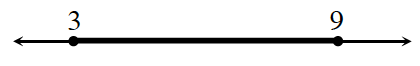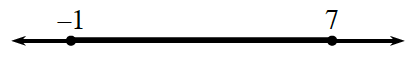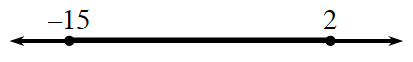### Home > GB8I > Chapter 7 Unit 8 > Lesson INT1: 7.1.6 > Problem7-71

7-71.

Each of these number lines shows a segment in bold. Determine the midpoint of the bold segment. Note that the diagrams are not drawn to scale.

The midpoint is the mean, or average. What is the mean of each of these pairs of numbers?

1.$\frac{\left(3+9\right)}{2}=6$

2.3.